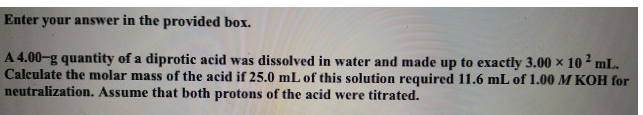Chemistry Practice Problems Diprotic Acid Practice Problems Solution: Enter your answer in the provided box.A 4.00-g qua...

# Solution: Enter your answer in the provided box.A 4.00-g quantity of a diprotic acid was dissolved in water and made up to exactly 3.00 x 102 mL. Calculate the molar mass of the acid if 25.0 mL of this solution required 11.6 mL of 1.00 M KOH for neutralization. Assume that both protons of the acid were titrated.

###### Problem

A 4.00-g quantity of a diprotic acid was dissolved in water and made up to exactly 3.00 x 102 mL. Calculate the molar mass of the acid if 25.0 mL of this solution required 11.6 mL of 1.00 M KOH for neutralization. Assume that both protons of the acid were titrated.View Complete Written Solution

Diprotic Acid

Diprotic Acid

#### Q. State whether each of the following statements is true or false. Justify your answer in each case.Sulfuric acid is a monoprotic acid.

Solved • Wed Oct 31 2018 12:47:00 GMT-0400 (EDT)

Diprotic Acid

#### Q. Complete the Ka2 expression for H2CO3 in an aqueous solution.

Solved • Tue Jun 19 2018 10:53:36 GMT-0400 (EDT)

Diprotic Acid

#### Q. What is the pH of a 0.310 M solution of H2SO4? Ka2 = 1.20 x 10^-2

Solved • Tue May 01 2018 09:40:37 GMT-0400 (EDT)

Diprotic Acid

#### Q. Which one of the following is a diprotic acid?a. sulfuric acidb. nitric acidc. barium hydroxided. chloric acid

Solved • Mon Apr 02 2018 10:49:49 GMT-0400 (EDT)